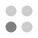• 5,337 Trophies Earned
• 276 Players Tracked
• 24 Total Trophies
• 226 Obtainable EXP
• 1,200 Points
• 198 Platinum Club
• 198 100% Club

### Pic-a-Pix Classic Trophies

•Collect all the trophies71.74%
•Solve a 5x5 puzzle99.64%
•Solve a 10x10 puzzle97.10%
•Solve a 10x10 puzzle without using an X96.74%
•Solve all the 10x10 puzzles84.06%
•Earn a medal for every 10x10 puzzle82.61%
•Solve a 15x15 puzzle88.41%
•Solve a 15x15 puzzle without using an X86.59%
•Solve all the 15x15 puzzles74.28%
•Earn a medal for every 15x15 puzzle73.91%
•Solve a 20x15 puzzle82.61%
•Solve a 20x15 puzzle without using an X81.88%
•Solve all the 20x15 puzzles72.46%
•Earn a medal for every 20x15 puzzle72.10%
•Solve a 20x20 puzzle81.88%
•Solve a 20x20 puzzle without using an X81.16%
•Solve all the 20x20 puzzles74.28%
•Earn a medal for every 20x20 puzzle73.19%
•Solve a 30x20 puzzle82.25%
•Solve a 30x20 puzzle without using an X81.16%
•Solve all the 30x20 puzzles76.81%
•Earn a medal for every 30x20 puzzle75.00%
•Solve every puzzle72.10%
•Earn a medal for every puzzle71.74%